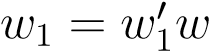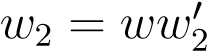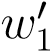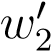1. Engineering
2. Computer Science
3. let be an alphabet given two words w1 and...

# Question: let be an alphabet given two words w1 and...

###### Question details

Let Σ be an alphabet. Given two words w1 and w2, a match between w1 and w2 is a word w satisfyingandfor someand. Given two languages L1 and L2, we use L1L2 to denote the set of matches between w1 and w2 for all w1L1,w2L2. Prove that if L1 and L2 are regular languages, then so is L1L2.

###### Solution by an expert tutor